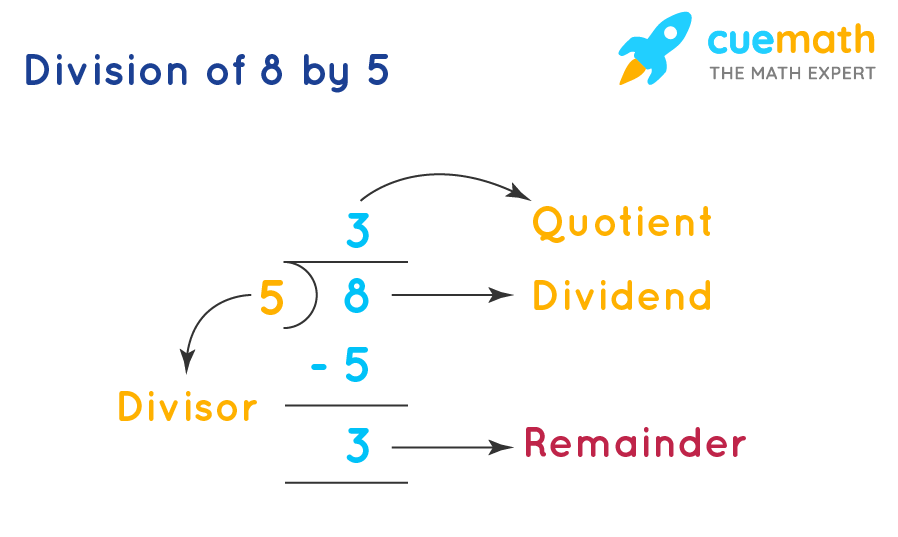# What Do We Call the Number We Are Divide Into?

## Question: What do we call the number we are divided into?

We will see the parts of a division problem.

## Answer: The numbers In the division are called Dividend, Divisor, Quotient and Remainder.

We will answer the question by taking one example.

## Explanation:

Consider the division 8 ÷ 5